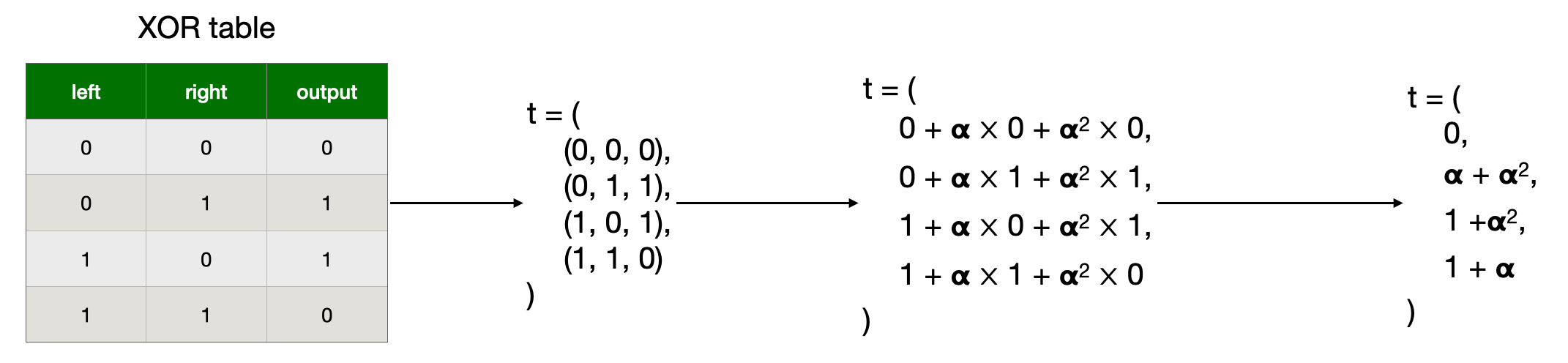allows us to check if witness values belong to a look up table. This is usualy useful for reducing the number of constraints needed for bit-wise operations. So in the rest of this document we’ll use the XOR table as an example.

ABY
000
011
101
110

First, let’s define some terms:

• lookup table: a table of values that means something, like the XOR table above
• joint lookup table: a table where cols have been joined together in a single col (with a challenge)
• table entry: a cell in a joint lookup table
• single lookup value: a value we’re trying to look up in a table
• joint lookup values: several values that have been joined together (with a challenge)

A joint lookup table looks like this, for some challenge :## Constraints

Computes the aggregation polynomial for maximum lookups per row, whose -th entry is the product of terms:

for .

• is the -th entry in the table
• is the -th lookup in the -th row of the witness

For every instance of a value in and , there is an instance of the same value in

is sorted in the same order as , increasing along the ‘snake-shape’

Whenever the same value is in and , that term in the denominator product becomes

this will cancel with the corresponding looked-up value in the witness

Whenever the values and differ, that term in the denominator product will cancel with some matching

because the sorting is the same in and .

There will be exactly the same number of these as the number of values in if only contains values from . After multiplying all of the values, all of the terms will have cancelled if is a sorting of and , and the final term will be because of the random choice of and , there is negligible probability that the terms will cancel if is not a sorting of and

But because of the snakify:

• we are repeating an element between cols, we need to check that it’s the same in a constraint
• we invert the direction, but that shouldn’t matter

## FAQ

• how do we do multiple lookups per row?
• how do we dismiss rows where there are no lookup?